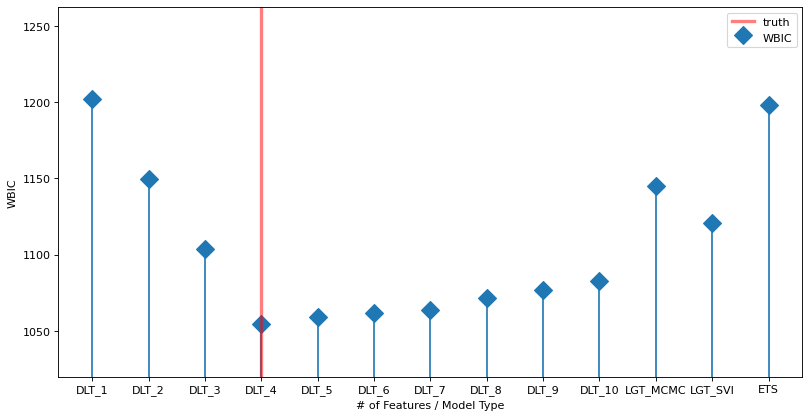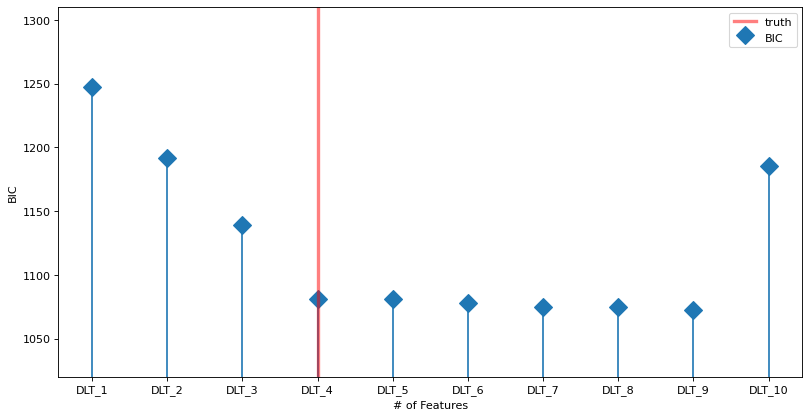# WBIC/BIC¶

This notebook gives a tutorial on how to use Watanabe-Bayesian information criterion (WBIC) and Bayesian information criterion (BIC) for feature selection (Watanabe, McElreath, and Vehtari). The WBIC or BIC is an information criterion. Similar to other criteria (AIC, DIC), the WBIC/BIC endeavors to find the most parsimonious model, i.e., the model that balances fit with complexity. In other words a model (or set of features) that optimizes WBIC/BIC should neither over nor under fit the available data.

In this tutorial a data set is simulated using the damped linear trend (DLT) model. This data set is then used to fit DLT models with varying number of features as well as a global local trend model (GLT), and a Error-Trend-Seasonal (ETS) model. The WBIC/BIC criteria is then show to find the true model.

Note that we recommend the use of WBIC for full Bayesian and SVI estimators and BIC for MAP estimator.

:

from datetime import timedelta

import pandas as pd
import numpy as np

import matplotlib.pyplot as plt
%matplotlib inline

import orbit
from orbit.models import DLT,ETS, KTRLite, LGT
from orbit.utils.simulation import make_trend, make_regression

:

print(orbit.__version__)

1.1.4


## Data Simulation¶

This block of code creates random data set (365 observations with 10 features) assuming a DLT model. Of the 10 features 5 are effective regressors; i.e., they are used in the true model to create the data.

As an exercise left to the user once you have run the code once try changing the NUM_OF_EFFECTIVE_REGRESSORS (line 2), the SERIES_LEN (line 3), and the SEED (line 4) to see how it effects the results.

:

NUM_OF_REGRESSORS = 10
NUM_OF_EFFECTIVE_REGRESSORS = 4
SERIES_LEN = 365
SEED = 1
# sample some coefficients
COEFS = np.random.default_rng(SEED).uniform(-1, 1, NUM_OF_EFFECTIVE_REGRESSORS)
trend = make_trend(SERIES_LEN, rw_loc=0.01, rw_scale=0.1)
x, regression, coefs = make_regression(series_len=SERIES_LEN, coefs=COEFS)

# combine trend and the regression
y = trend + regression
y = y - y.min()

x_extra = np.random.normal(0, 1, (SERIES_LEN, NUM_OF_REGRESSORS - NUM_OF_EFFECTIVE_REGRESSORS))
x = np.concatenate([x, x_extra], axis=-1)

x_cols = [f"x{x}" for x in range(1, NUM_OF_REGRESSORS + 1)]
response_col = "y"
dt_col = "date"
obs_matrix = np.concatenate([y.reshape(-1, 1), x], axis=1)
# make a data frame for orbit inputs
df = pd.DataFrame(obs_matrix, columns=[response_col] + x_cols)
# make some dummy date stamp
dt = pd.date_range(start='2016-01-04', periods=SERIES_LEN, freq="1W")
df['date'] = dt

:

print(df.shape)

(365, 12)
y        x1        x2        x3        x4        x5        x6  \
0  4.426242  0.172792  0.000000  0.165219 -0.000000 -0.309807 -2.622861
1  5.580432  0.452678  0.223187 -0.000000  0.290559  1.357638  0.245350
2  5.031773  0.182286  0.147066  0.014211  0.273356  1.366471  0.360691
3  3.264027 -0.368227 -0.081455 -0.241060  0.299423 -0.078815 -0.584712
4  5.246511  0.019861 -0.146228 -0.390954 -0.128596  0.052695 -1.017173

x7        x8        x9       x10       date
0 -0.606916 -1.439585  0.053856 -0.237528 2016-01-10
1  2.236552 -0.810457 -0.728617 -1.045430 2016-01-17
2 -0.315396  0.063182  0.543401  1.077713 2016-01-24
3 -1.537894  1.397256  0.380956  0.130842 2016-01-31
4 -1.615387  0.730674  0.934285  1.538906 2016-02-07


## WBIC¶

In this section, we use DLT model as an example. Different DLT models (the number of features used changes) are fitted and their WBIC values are calculated respectively.

:

%%time
WBIC_ls = []
for k in range(1, NUM_OF_REGRESSORS + 1):
regressor_col = x_cols[:k]
dlt_mod = DLT(
response_col=response_col,
date_col=dt_col,
regressor_col=regressor_col,
seed=2022,
# fixing the smoothing parameters to learn regression coefficients more effectively
level_sm_input=0.01,
slope_sm_input=0.01,
num_warmup=4000,
num_sample=4000,
)
WBIC_temp = dlt_mod.fit_wbic(df=df)
print("WBIC value with {:d} regressors: {:.3f}".format(k, WBIC_temp))
print('------------------------------------------------------------------')
WBIC_ls.append(WBIC_temp)

2023-01-20 22:37:37 - orbit - INFO - Sampling (PyStan) with chains: 4, cores: 8, temperature: 5.900, warmups (per chain): 1000 and samples(per chain): 1000.



2023-01-20 22:37:49 - orbit - INFO - Sampling (PyStan) with chains: 4, cores: 8, temperature: 5.900, warmups (per chain): 1000 and samples(per chain): 1000.

WBIC value with 1 regressors: 1201.735
------------------------------------------------------------------



2023-01-20 22:38:00 - orbit - INFO - Sampling (PyStan) with chains: 4, cores: 8, temperature: 5.900, warmups (per chain): 1000 and samples(per chain): 1000.

WBIC value with 2 regressors: 1150.356
------------------------------------------------------------------



2023-01-20 22:38:12 - orbit - INFO - Sampling (PyStan) with chains: 4, cores: 8, temperature: 5.900, warmups (per chain): 1000 and samples(per chain): 1000.

WBIC value with 3 regressors: 1103.973
------------------------------------------------------------------



2023-01-20 22:38:24 - orbit - INFO - Sampling (PyStan) with chains: 4, cores: 8, temperature: 5.900, warmups (per chain): 1000 and samples(per chain): 1000.

WBIC value with 4 regressors: 1054.947
------------------------------------------------------------------



2023-01-20 22:38:36 - orbit - INFO - Sampling (PyStan) with chains: 4, cores: 8, temperature: 5.900, warmups (per chain): 1000 and samples(per chain): 1000.

WBIC value with 5 regressors: 1060.966
------------------------------------------------------------------



2023-01-20 22:38:48 - orbit - INFO - Sampling (PyStan) with chains: 4, cores: 8, temperature: 5.900, warmups (per chain): 1000 and samples(per chain): 1000.

WBIC value with 6 regressors: 1066.917
------------------------------------------------------------------



2023-01-20 22:39:00 - orbit - INFO - Sampling (PyStan) with chains: 4, cores: 8, temperature: 5.900, warmups (per chain): 1000 and samples(per chain): 1000.

WBIC value with 7 regressors: 1073.725
------------------------------------------------------------------



2023-01-20 22:39:12 - orbit - INFO - Sampling (PyStan) with chains: 4, cores: 8, temperature: 5.900, warmups (per chain): 1000 and samples(per chain): 1000.

WBIC value with 8 regressors: 1079.453
------------------------------------------------------------------



2023-01-20 22:39:24 - orbit - INFO - Sampling (PyStan) with chains: 4, cores: 8, temperature: 5.900, warmups (per chain): 1000 and samples(per chain): 1000.

WBIC value with 9 regressors: 1085.690
------------------------------------------------------------------


WBIC value with 10 regressors: 1091.948
------------------------------------------------------------------
CPU times: user 22.8 s, sys: 1.14 s, total: 24 s
Wall time: 1min 58s


It is also interesting to see if WBIC can distinguish between model types; not just do feature selection for a given type of model. To that end the next block fits an LGT and ETS model to the data; the WBIC values for both models are then calculated.

Note that WBIC is supported for both the ‘stan-mcmc’ and ‘pyro-svi’ estimators. Currently only the LGT model has both. Thus WBIC is calculated for LGT for both estimators.

:

%%time
lgt = LGT(response_col=response_col,
date_col=dt_col,
regressor_col=regressor_col,
seasonality=52,
estimator='stan-mcmc',
seed=8888)
WBIC_lgt_mcmc = lgt.fit_wbic(df=df)
print("WBIC value for LGT model (stan MCMC): {:.3f}".format(WBIC_lgt_mcmc))

lgt = LGT(response_col=response_col,
date_col=dt_col,
regressor_col=regressor_col,
seasonality=52,
estimator='pyro-svi',
seed=8888)
WBIC_lgt_pyro = lgt.fit_wbic(df=df)
print("WBIC value for LGT model (pyro SVI): {:.3f}".format(WBIC_lgt_pyro))

ets = ETS(
response_col=response_col,
date_col=dt_col,
seed=2020,
# fixing the smoothing parameters to learn regression coefficients more effectively
level_sm_input=0.01,
)

WBIC_ets = ets.fit_wbic(df=df)
print("WBIC value for ETS model: {:.3f}".format(WBIC_ets))

WBIC_ls.append(WBIC_lgt_mcmc)
WBIC_ls.append(WBIC_lgt_pyro)
WBIC_ls.append(WBIC_ets)

2023-01-20 22:39:36 - orbit - INFO - Sampling (PyStan) with chains: 4, cores: 8, temperature: 5.900, warmups (per chain): 225 and samples(per chain): 25.



2023-01-20 22:39:37 - orbit - INFO - Using SVI (Pyro) with steps: 301, samples: 100, learning rate: 0.1, learning_rate_total_decay: 1.0 and particles: 100.


WBIC value for LGT model (stan MCMC): 1137.127

2023-01-20 22:39:37 - orbit - INFO - step    0 loss = 313.26, scale = 0.11492
INFO:orbit:step    0 loss = 313.26, scale = 0.11492
2023-01-20 22:39:45 - orbit - INFO - step  100 loss = 116.76, scale = 0.50764
INFO:orbit:step  100 loss = 116.76, scale = 0.50764
2023-01-20 22:39:54 - orbit - INFO - step  200 loss = 116.66, scale = 0.50072
INFO:orbit:step  200 loss = 116.66, scale = 0.50072
2023-01-20 22:40:02 - orbit - INFO - step  300 loss = 117.05, scale = 0.50493
INFO:orbit:step  300 loss = 117.05, scale = 0.50493
2023-01-20 22:40:02 - orbit - INFO - Sampling (PyStan) with chains: 4, cores: 8, temperature: 5.900, warmups (per chain): 225 and samples(per chain): 25.
INFO:orbit:Sampling (PyStan) with chains: 4, cores: 8, temperature: 5.900, warmups (per chain): 225 and samples(per chain): 25.

WBIC value for LGT model (pyro SVI): 1130.131


WBIC value for ETS model: 1201.702
CPU times: user 23.3 s, sys: 3.89 s, total: 27.2 s
Wall time: 26.5 s


The plot below shows the WBIC vs the number of features / model type (blue line). The true model is indicated by the vertical red line. The horizontal gray line shows the minimum (optimal) value. The minimum is at the true value.

:

labels = ["DLT_{}".format(x) for x in range(1, NUM_OF_REGRESSORS + 1)] + ['LGT_MCMC', 'LGT_SVI','ETS']
fig, ax = plt.subplots(1, 1,figsize=(12, 6), dpi=80)
markerline, stemlines, baseline = ax.stem(
np.arange(len(labels)), np.array(WBIC_ls), label='WBIC', markerfmt='D')
baseline.set_color('none')
markerline.set_markersize(12)
ax.set_ylim(1020, )
ax.set_xticks(np.arange(len(labels)))
ax.set_xticklabels(labels)
# because list type is mixed index from 1;
ax.axvline(x=NUM_OF_EFFECTIVE_REGRESSORS - 1, color='red', linewidth=3, alpha=0.5, linestyle='-', label='truth')
ax.set_ylabel("WBIC")
ax.set_xlabel("# of Features / Model Type")
ax.legend();## BIC¶

In this section, we use DLT model as an example. Different DLT models (the number of features used changes) are fitted and their BIC values are calculated respectively.

:

%%time
BIC_ls = []
for k in range(0, NUM_OF_REGRESSORS):
regressor_col = x_cols[:k + 1]
dlt_mod = DLT(
estimator='stan-map',
response_col=response_col,
date_col=dt_col,
regressor_col=regressor_col,
seed=2022,
# fixing the smoothing parameters to learn regression coefficients more effectively
level_sm_input=0.01,
slope_sm_input=0.01,
)
dlt_mod.fit(df=df)
BIC_temp = dlt_mod.get_bic()
print("BIC value with {:d} regressors: {:.3f}".format(k + 1, BIC_temp))
print('------------------------------------------------------------------')
BIC_ls.append(BIC_temp)

2023-01-20 22:40:02 - orbit - INFO - Optimizing (PyStan) with algorithm: LBFGS.
INFO:orbit:Optimizing (PyStan) with algorithm: LBFGS.
2023-01-20 22:40:03 - orbit - INFO - Optimizing (PyStan) with algorithm: LBFGS.
INFO:orbit:Optimizing (PyStan) with algorithm: LBFGS.
2023-01-20 22:40:03 - orbit - INFO - Optimizing (PyStan) with algorithm: LBFGS.
INFO:orbit:Optimizing (PyStan) with algorithm: LBFGS.
2023-01-20 22:40:03 - orbit - INFO - Optimizing (PyStan) with algorithm: LBFGS.
INFO:orbit:Optimizing (PyStan) with algorithm: LBFGS.

BIC value with 1 regressors: 1247.444
------------------------------------------------------------------
BIC value with 2 regressors: 1191.902
------------------------------------------------------------------
BIC value with 3 regressors: 1139.408
------------------------------------------------------------------
BIC value with 4 regressors: 1079.642
------------------------------------------------------------------

2023-01-20 22:40:03 - orbit - INFO - Optimizing (PyStan) with algorithm: LBFGS.
INFO:orbit:Optimizing (PyStan) with algorithm: LBFGS.
2023-01-20 22:40:03 - orbit - INFO - Optimizing (PyStan) with algorithm: LBFGS.
INFO:orbit:Optimizing (PyStan) with algorithm: LBFGS.
2023-01-20 22:40:03 - orbit - INFO - Optimizing (PyStan) with algorithm: LBFGS.
INFO:orbit:Optimizing (PyStan) with algorithm: LBFGS.

BIC value with 5 regressors: 1082.568
------------------------------------------------------------------
BIC value with 6 regressors: 1082.299
------------------------------------------------------------------

2023-01-20 22:40:03 - orbit - INFO - Optimizing (PyStan) with algorithm: LBFGS.
INFO:orbit:Optimizing (PyStan) with algorithm: LBFGS.
2023-01-20 22:40:03 - orbit - INFO - Optimizing (PyStan) with algorithm: LBFGS.
INFO:orbit:Optimizing (PyStan) with algorithm: LBFGS.
2023-01-20 22:40:03 - orbit - INFO - Optimizing (PyStan) with algorithm: LBFGS.
INFO:orbit:Optimizing (PyStan) with algorithm: LBFGS.

BIC value with 7 regressors: 1082.001
------------------------------------------------------------------
BIC value with 8 regressors: 1081.729
------------------------------------------------------------------
BIC value with 9 regressors: 1079.434
------------------------------------------------------------------
BIC value with 10 regressors: 1188.806
------------------------------------------------------------------
CPU times: user 416 ms, sys: 221 ms, total: 636 ms
Wall time: 806 ms


The plot below shows the BIC vs the number of features (blue line). The true model is indicated by the vertical red line. The horizontal gray line shows the minimum (optimal) value. The minimum is at the true value.

:

labels = ["DLT_{}".format(x) for x in range(1, NUM_OF_REGRESSORS + 1)]
fig, ax = plt.subplots(1, 1,figsize=(12, 6), dpi=80)
markerline, stemlines, baseline = ax.stem(
np.arange(len(labels)), np.array(BIC_ls), label='BIC', markerfmt='D')
baseline.set_color('none')
markerline.set_markersize(12)
ax.set_ylim(1020, )
ax.set_xticks(np.arange(len(labels)))
ax.set_xticklabels(labels)
# because list type is mixed index from 1;
ax.axvline(x=NUM_OF_EFFECTIVE_REGRESSORS - 1, color='red', linewidth=3, alpha=0.5, linestyle='-', label='truth')
ax.set_ylabel("BIC")
ax.set_xlabel("# of Features")
ax.legend();